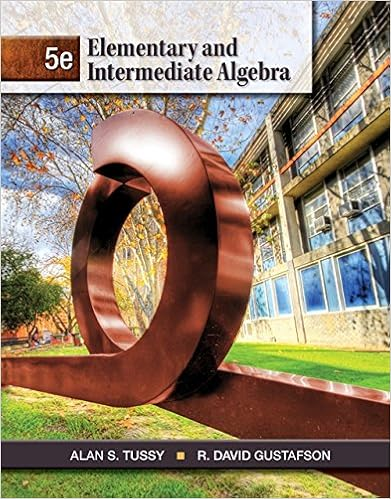# Page 1 of 12 math 1070q sample final b graph the

• Test Prep
• 13
• 100% (2) 2 out of 2 people found this document helpful

This preview shows page 2 - 7 out of 13 pages.

##### We have textbook solutions for you!
The document you are viewing contains questions related to this textbook.The document you are viewing contains questions related to this textbook.
Chapter 11 / Exercise 77
Elementary and Intermediate Algebra
Tussy/GustafsonExpert Verified
Page 1 of 12
##### We have textbook solutions for you!
The document you are viewing contains questions related to this textbook.The document you are viewing contains questions related to this textbook.
Chapter 11 / Exercise 77
Elementary and Intermediate Algebra
Tussy/GustafsonExpert Verified
Math 1070QSample Final(b) Graph the feasible region and find its corner points.(c) Determine the number of bowls and trays to be produced to maximize profit.Page 2 of 12
Math 1070QSample Final3. A car dealership tracks the number of cars sold every week for several weeks. Let the randomvariableXrepresent the number of cars sold every week.(a) Complete the probability distribution table below.
(b) Find the expected value E(X).(c) Find the variance Var(X).Page 3 of 12
•••